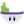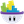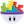#### Howdy, Stranger!

It looks like you're new here. If you want to get involved, click one of these buttons!

# Collision Issue

Posts: 55Member, PROSo I have a game where I need a ball to bounce off of a wall, but over time the ball will eventually end up going either perfectly horizontal or perfectly vertical. How can I make it so that it is pretty much always going to be moving one way or another (prevent it from getting stuck in the vert/horizontal). I tried making a rule if linear velocity x is 0 then change velocity random angle but it doesn't work?

• Posts: 1,182MemberIn ball

When collide with wall.
Change self velocity x to velocityx *-1
Change self velocity y to velocity. *-1

Try that and see if it bounces better.

Could be a friction thing. Change that to 0 in each.

• London, UK.Posts: 12,822Memberedited May 2017

@Toque said:
In ball

When collide with wall.
Change self velocity x to velocityx *-1
Change self velocity y to velocity. *-1

That's not going to work !

If the ball is heading, for example, at 45° (between 1 and 2 on a clock face) towards the righthand wall of a square boundary, then when it collides the Y velocity (which should continue to go upwards) will reverse and go downwards.

Hope that makes sense.

• Posts: 1,182MemberHmmm.

I think you idea would work but need a range.
If velocity x is between 0 and 20 add 30
If veolicity x is between 0 and -20 minus 30.

• London, UK.Posts: 12,822Memberedited May 2017

@Toque said:
Hmmm.

I think you idea would work but need a range.
If velocity x is between 0 and 20 add 30
If veolicity x is between 0 and -20 minus 30.

Using the same example above (a ball travelling at 45° towards a vertical wall on its right) . . . if the ball were to hit the wall at an X velocity of 15 . . . then a rule saying [If velocity x is between 0 and 20 then add 30] would see the ball's X velocity triple in speed (from 15 to 45) and continue to travel right, rather than bounce off the wall and continue to move left at the same relative speed (in this case -15).

• Posts: 1,182Memberedited May 2017

Starting to sink in. I had this same problem. Trying to remember solution. I'm close.

I think I got it. Can't do demo till later.

• Posts: 55Member, PRO@Socks said:

@Toque said:
Hmmm.

I think you idea would work but need a range.
If velocity x is between 0 and 20 add 30
If veolicity x is between 0 and -20 minus 30.

Using the same example above (a ball travelling at 45° towards a vertical wall on its right) . . . if the ball were to hit the wall at an X velocity of 15 . . . then a rule saying [If velocity x is between 0 and 20 then add 30] would see the ball's X velocity triple in speed (from 15 to 45) and continue to travel right, rather than bounce off the wall and continue to move left at the same relative speed (in this case -15).

@Toque said:
Starting to sink in. I had this same problem. Trying to remember solution. I'm close.

I think I got it. Can't do demo till later.

Thanks both of you guys! Friction is Zero on both, Bounce is 2, and density is 1. Why wont the collision rules in GS work by themselves?

• Posts: 1,483Memberedited May 2017

Edit: just realised this was answered• Posts: 1,182MemberUsing the same example above (a ball t

Thanks both of you guys! Friction is Zero on both, Bounce is 2, and density is 1. Why wont the collision rules in GS work by themselves?

It is working. Too well. But not always as our brain thinks it should.